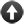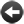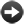§15.15. The parts of a number specification

We often need to break up a number specification into its pieces. For instance, suppose we want to know the dollars part of \$1.99? We can do this by naming the parts:

A monetary value is a kind of value. \$1.99 specifies a monetary value with parts dollars and cents.

We can now find the relevant parts like so. Suppose that "sum" is a monetary value. Then:

dollars part of sum
cents part of sum

are both numbers, so for instance we can

say "Looks like around [dollars part of sum in words] dollar[s]."

We can also go the other way:

monetary value with dollars part 4 cents part 72

produces the monetary value \$4.72. (Note the lack of commas or "and"s, and that the parts have to be given in the right order.) This is really intended to be useful when we manipulate such values in unusual ways:

An aspect ratio is a kind of value. 16:20 specifies an aspect ratio with parts width and height.

To decide which aspect ratio is the wider version of (AR - an aspect ratio):
let W be the width part of AR multiplied by 2;
let H be the height part of AR;
let the wider ratio be the aspect ratio with width part W height part H;
decide on the wider ratio.

Declaring the parts of a number specification individually also enables us to tack one or more options onto any of the parts:

A monetary value is a kind of value. \$1.99 specifies a monetary value with parts dollars and cents (optional, preamble optional).

This declares that the "cents" part is optional - it will be 0 if not specified - and that if omitted, the non-numeric "preamble" before it should also be omitted. Thus "\$3" is now valid and equivalent to "\$3.00": indeed it will be the preferred form when Inform prints out a monetary value which is an exact number of dollars. If we had said that "cents" was optional, but not said that the preamble was optional, then "\$3." would have been the form - which is less satisfactory.

There is only one other option: "without leading zeros", as in the following.

An aspect ratio is a kind of value. 16:20 specifies an aspect ratio with parts width and height (without leading zeros).

This ensures that when the ratio 4:3 is printed, it will be printed as "4:3" and not "4:03" as would otherwise happen.Start of Chapter 15: Numbers and EquationsBack to §15.14. Notations including more than one numberOnward to §15.16. Understanding specified numbers